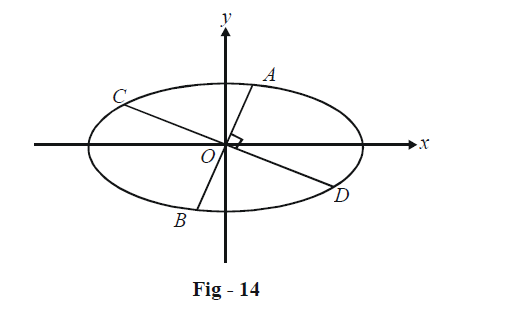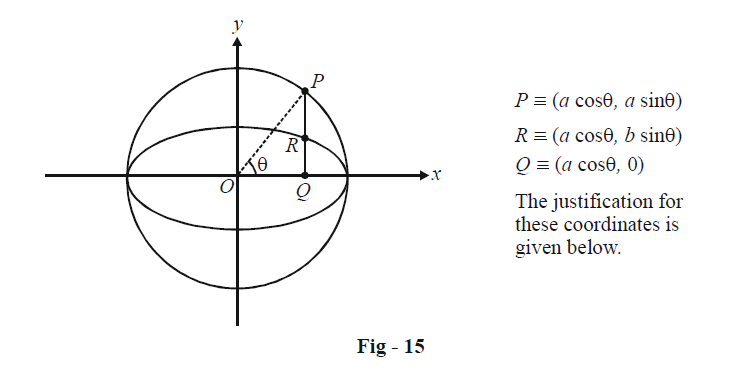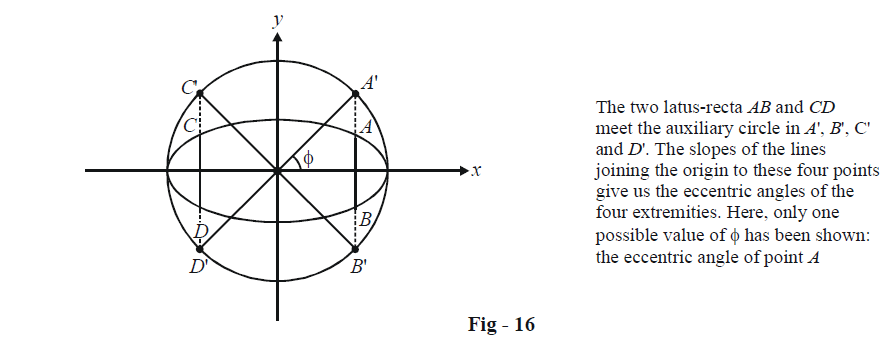# Basic Examples On Ellipses Set-3

Go back to  'Ellipse'

Example - 6

Show that the sum of the reciprocals of the squares of any two diameters of an ellipse which are at right angles to one another is a constant.

Solution: By a diameter of an ellipse, we mean any chord which passes through its centre.

Let \begin{align}\frac{{{x^2}}}{{{a^2}}} + \frac{{{y^2}}}{{{b^2}}} = 1\end{align} be the ellipse and let AB and CD be any two diameters of the ellipse perpendicular to each other.Since AB and CD are diameters, we can assume AO = OB = r1, and CO = OD = r2. Also, if the slope of AB is given by $$\theta$$ than that of CD is obviously \begin{align}\frac{\pi }{2} + \theta .\end{align}

Thus, we get the co-ordinates of A, B, C and D as

\begin{align}&A,\,\,B \equiv \pm ({r_1}\cos {\theta _1},\,{r_1}\sin \theta ){\rm{ }}\qquad C,D \equiv \pm \left( {{r_2}\cos \left( {\frac{\pi }{2} + \theta } \right),\,\,{r_2}\sin \left( {\frac{\pi }{2} + \theta } \right)} \right)\\&{\rm{\; }}\qquad\qquad\qquad\qquad \qquad\qquad\qquad\qquad= \pm ( - {r_2}\sin \theta ,\,\,{r_2}\cos \theta )\end{align}

These coordinates must satisfy the equation of the ellipse; we therefore obtain :

\begin{align}&\frac{{r_1^2{{\cos }^2}\theta }}{{{a^2}}} + \frac{{r_1^2{{\sin }^2}\theta }}{{{b^2}}} = 1\;and\;\frac{{r_2^2{{\sin }^2}\theta }}{{{a^2}}} + \frac{{r_2^2{{\cos }^2}\theta }}{{{b^2}}} = 1\\&\Rightarrow \qquad \frac{1}{{r_1^2}} = \frac{{{{\cos }^2}\theta }}{{{a^2}}} + \frac{{{{\sin }^2}\theta }}{{{b^2}}}\;and\;\frac{1}{{r_2^2}} = \frac{{{{\sin }^2}\theta }}{{{a^2}}} + \frac{{{{\cos }^2}\theta }}{{{b^2}}}\end{align}

Adding these two relations, we have

\begin{align}&\frac{1}{{r_1^2}} + \frac{1}{{r_2^2}} = \frac{1}{{{a^2}}} + \frac{1}{{{b^2}}}\\& \Rightarrow \qquad \frac{1}{{{{(2{r_1})}^2}}} + \frac{1}{{{{(2{r_2})}^2}}} = \frac{1}{{4{a^2}}} + \frac{1}{{4{b^2}}}\\&\Rightarrow\qquad \frac{1}{{A{B^2}}} + \frac{1}{{C{D^2}}} = \frac{1}{{4{a^2}}} + \frac{1}{{4{b^2}}}\left( {a{\rm{ \;}}constant} \right)\end{align}

This proves the assertion stated in the question.

## AUXILIARY CIRCLE

Example – 7

Let $$\frac{{{x^2}}}{{{a^2}}} + \frac{{{y^2}}}{{{b^2}}} = 1$$ be an ellipse. Assume a > b. A circle is described on the major axis of this ellipse as diameter. From any point P on this circle, a perpendicular PQ is dropped onto the major axis of the ellipse. Show that PQ will always be divided in a fixed ratio by the ellipse.

Solution:As is evident, the radius of this circle, called the auxiliary circle of the ellipse, is a, so that its equation is

${x^2} + {y^2} = {a^2}$

Now any point P on this circle can be taken in parametric form as $$P \equiv (a\cos \theta ,\,a\sin \theta )$$ where $$\theta$$ is the angle that OP makes with the horizontal.

To evaluate the y-coordinate of R, we substitute $$x = a\cos \theta$$ in the equation of the ellipse:

\begin{align}&\frac{{{a^2}{{\cos }^2}\theta }}{{{a^2}}} + \frac{{{y^2}}}{{{b^2}}} = 1\\&\Rightarrow y = b\sin \theta\end{align}

Thus, R is the point $$(a\cos \theta ,\,b\sin \theta )$$ while Q is simply $$(a\cos \theta ,\,0).$$ We now see that

$\frac{{PR}}{{RQ}} = \frac{{a\sin \theta - b\sin \theta }}{{b\sin \theta }} = \frac{a}{b} - 1$

which is independent of $$\theta$$ proving the stated assertion.

There is one significant fact that we can learn about the ellipse $$\frac{{{x^2}}}{{{a^2}}} + \frac{{{y^2}}}{{{b^2}}} = 1.$$ We obtained the coordinates of R as$$(a\cos \theta ,\,b\sin \theta ).$$ This tells us that an alternative way to specify an ellipse is in terms of a parameter $$\theta$$ :

$\boxed{x = a\cos \theta ,\,\,y = b\sin \theta }$

This is referred to as the parametric form of the ellipse $$\frac{{{x^2}}}{{{a^2}}} + \frac{{{y^2}}}{{{b^2}}} = 1.$$$$\theta$$ is called the eccentric angle of the point $$(x,\,y) \equiv (a\cos \theta ,\,\,b\sin \theta ).$$ It is important to note that $$\theta$$ is not the angle that $$(a\cos \theta ,\,\,b\sin \theta )$$ makes with the horizontal; it is the angle which the corresponding point on the auxiliary circle makes with the horizontal. Depending on what value $$\theta$$ takes in the range$$[0,\,2\pi )$$$$({\rm{or ( - }}\pi {\rm{, }}\pi ]),$$the parametric form $$(a\cos \theta ,\,b\sin \theta )$$ gives us different points on the circumference of the ellipse.

The point $$(a\cos \theta ,\,b\sin \theta )$$ in sometimes simply referred to as the point $$\theta$$

Example - 8

What are the eccentric angles of the extremities of the latus-recta in the ellipse $$\frac{{{x^2}}}{{{a^2}}} + \frac{{{y^2}}}{{{b^2}}} = 1\,\,?$$

Solution: As discussed earlier, the co-ordinates of the end-points of the latus-recta are $$\left( { \pm ae,\,\, \pm \frac{{{b^2}}}{a}} \right).$$ Thus, if we assume the required eccentric angle to be $$\phi ,$$we have

\begin{align}&a\cos \phi = \pm ae\\&b\sin \phi = \pm \frac{{{b^2}}}{a}\end{align}

This gives us four values of $$\phi ,$$ given by

$\tan \phi = \pm \frac{b}{{ae}}$

corresponding to the four extremities of the two latus-recta: